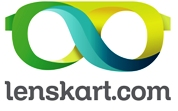Charged Up Array
Tag(s):

## Arrays, Data Structures, Easy, One-dimensional

Problem
Editorial
Analytics

You are given with an array $A$ of size $N$.An element $A_i$ is said to be charged if its value($A_i$) is greater than or equal to $K_i$. $K_i$ is the total number of subsets of array $A$, that consist of element $A_i$
Total charge value of the array is defined as summation of all charged elements present in the array mod $10^9+7$.
Your task is to output the total charge value of the given array $A$.

INPUT FORMAT:

The first line of input contains number of test cases $T$.
The first line of each test case consists of $N$, the size of the array.
Next line contains $N$ space-separated integers corresponding to each element $A_i$.

OUTPUT FORMAT:

For each test case, output a single number corresponding to total charge value of the given array.

CONSTRAINTS:
$1 \le T \le100\\ 1 \le N \le 10^5\\ 0 \le A_i \le 10^{18}\\$

SAMPLE INPUT
2
3
3 4 5
2
1 6
SAMPLE OUTPUT
9
6
Explanation

CASE 1:
Possible subsets are: {3}, {4}, {5}, {3,4}, {3,5}, {4,5}, {3,4,5}, {}.
Element 3 is present in 4 subsets. As 3<4, it is not charged.
Element 4 is present in 4 subsets. As 4>=4, it is charged.
Element 5 is present in 4 subsets. As 5>=4, it is charged.
Total charge=4+5=9

CASE 2:
Possible subsets are: {1}, {6}, {1,6}, {}.
Element 1 is present in 2 subsets. As 1<2, it is not charged.
Element 6 is present in 2 subsets. As 6>=2, it is charged.
Total charge=6

Time Limit: 2.0 sec(s) for each input file.
Memory Limit: 256 MB
Source Limit: 1024 KB
Marking Scheme: Marks are awarded when all the testcases pass.
Allowed Languages: Bash, C, C++, C++14, Clojure, C#, D, Erlang, F#, Go, Groovy, Haskell, Java, Java 8, JavaScript(Rhino), JavaScript(Node.js), Julia, Kotlin, Lisp, Lisp (SBCL), Lua, Objective-C, OCaml, Octave, Pascal, Perl, PHP, Python, Python 3, R(RScript), Racket, Ruby, Rust, Scala, Swift, Swift-4.1, TypeScript, Visual Basic

## CODE EDITOR

Initializing Code Editor...

## This Problem was Asked inChallenge Name

Lenskart SDE1 & SDE2 Hiring Challenge

OTHER PROBLEMS OF THIS CHALLENGE
• Algorithms > Searching
• Data Structures > Hash Tables